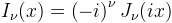Computes the regular modified cylindrical Bessel function.

Namespace:  Meta.Numerics.Functions
Assembly:  Meta.Numerics (in Meta.Numerics.dll) Version: 4.1.4Syntax
```public static double ModifiedBesselI(
double nu,
double x
)```

#### Parameters

nu
Type: SystemDouble
The order parameter.
x
Type: SystemDouble
The argument, which must be non-negative.

#### Return Value

Type: Double
The value of Iν(x).Exceptions
ExceptionCondition
ArgumentOutOfRangeExceptionx is negative.Remarks

The modified Bessel functions appear as the solutions of hyperbolic differential equations with cylindrical or circular symmetry, for example the conduction of heat through a cylindrical pipe.

The regular modified Bessel functions are related to the Bessel functions with pure imaginary arguments.The regular modified Bessel functions increase monotonically and exponentially from the origin.

Because they increase exponentially, this function overflows for even moderately large arguments. In this regeime, you can still obtain the value of the scaled modified e-x Iν(x) by calling ScaledModifiedBesselI(Double, Double).See Also